Download Presentation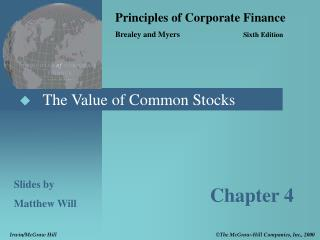Slides by Matthew Will

# Slides by Matthew Will - PowerPoint PPT Presentation

Principles of Corporate Finance Brealey and Myers Sixth Edition. The Value of Common Stocks. Slides by Matthew Will. Chapter 4. Irwin/McGraw Hill. The McGraw-Hill Companies, Inc., 2000. Topics Covered. How To Value Common Stock Capitalization Rates Stock Prices and EPSI am the owner, or an agent authorized to act on behalf of the owner, of the copyrighted work described.
Download Presentation## Slides by Matthew Will

An Image/Link below is provided (as is) to download presentation

Download Policy: Content on the Website is provided to you AS IS for your information and personal use and may not be sold / licensed / shared on other websites without getting consent from its author.While downloading, if for some reason you are not able to download a presentation, the publisher may have deleted the file from their server.

- - - - - - - - - - - - - - - - - - - - - - - - - - E N D - - - - - - - - - - - - - - - - - - - - - - - - - -
Presentation Transcript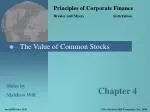Principles of Corporate Finance

Brealey and Myers Sixth Edition

• The Value of Common Stocks

Slides by

Matthew Will

Chapter 4

Irwin/McGraw Hill

• The McGraw-Hill Companies, Inc., 2000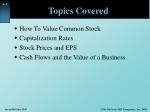Topics Covered
• How To Value Common Stock
• Capitalization Rates
• Stock Prices and EPS
• Cash Flows and the Value of a Business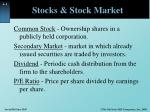Stocks & Stock Market

Common Stock - Ownership shares in a publicly held corporation.

Secondary Market - market in which already issued securities are traded by investors.

Dividend - Periodic cash distribution from the firm to the shareholders.

P/E Ratio - Price per share divided by earnings per share.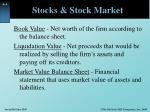Stocks & Stock Market

Book Value - Net worth of the firm according to the balance sheet.

Liquidation Value - Net proceeds that would be realized by selling the firm’s assets and paying off its creditors.

Market Value Balance Sheet - Financial statement that uses market value of assets and liabilities.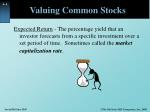Valuing Common Stocks

Expected Return- The percentage yield that an investor forecasts from a specific investment over a set period of time. Sometimes called the market capitalization rate.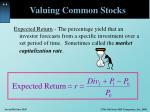Valuing Common Stocks

Expected Return- The percentage yield that an investor forecasts from a specific investment over a set period of time. Sometimes called the market capitalization rate.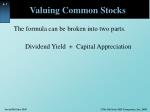Valuing Common Stocks

The formula can be broken into two parts.

Dividend Yield + Capital Appreciation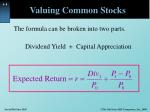Valuing Common Stocks

The formula can be broken into two parts.

Dividend Yield + Capital Appreciation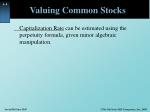Valuing Common Stocks

Capitalization Rate can be estimated using the perpetuity formula, given minor algebraic manipulation.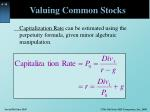Valuing Common Stocks

Capitalization Rate can be estimated using the perpetuity formula, given minor algebraic manipulation.Valuing Common Stocks

Return MeasurementsValuing Common Stocks

Dividend Discount Model - Computation of today’s stock price which states that share value equals the present value of all expected future dividends.Valuing Common Stocks

Dividend Discount Model - Computation of today’s stock price which states that share value equals the present value of all expected future dividends.

H - Time horizon for your investment.Valuing Common Stocks

Example

Current forecasts are for XYZ Company to pay dividends of \$3, \$3.24, and \$3.50 over the next three years, respectively. At the end of three years you anticipate selling your stock at a market price of \$94.48. What is the price of the stock given a 12% expected return?Valuing Common Stocks

Example

Current forecasts are for XYZ Company to pay dividends of \$3, \$3.24, and \$3.50 over the next three years, respectively. At the end of three years you anticipate selling your stock at a market price of \$94.48. What is the price of the stock given a 12% expected return?Valuing Common Stocks

If we forecast no growth, and plan to hold out stock indefinitely, we will then value the stock as a PERPETUITY.Valuing Common Stocks

If we forecast no growth, and plan to hold out stock indefinitely, we will then value the stock as a PERPETUITY.

Assumes all earnings are paid to shareholders.Valuing Common Stocks

Constant Growth DDM - A version of the dividend growth model in which dividends grow at a constant rate (Gordon Growth Model).Valuing Common Stocks

Example- continued

If the same stock is selling for \$100 in the stock market, what might the market be assuming about the growth in dividends?

The market is assuming the dividend will grow at 9% per year, indefinitely.Valuing Common Stocks
• If a firm elects to pay a lower dividend, and reinvest the funds, the stock price may increase because future dividends may be higher.

Payout Ratio - Fraction of earnings paid out as dividends

Plowback Ratio - Fraction of earnings retained by the firm.Valuing Common Stocks

Growth can be derived from applying the return on equity to the percentage of earnings plowed back into operations.

g = return on equity X plowback ratioValuing Common Stocks

Example

Our company forecasts to pay a \$5.00 dividend next year, which represents 100% of its earnings. This will provide investors with a 12% expected return. Instead, we decide to plow back 40% of the earnings at the firm’s current return on equity of 20%. What is the value of the stock before and after the plowback decision?Valuing Common Stocks

Example

Our company forecasts to pay a \$5.00 dividend next year, which represents 100% of its earnings. This will provide investors with a 12% expected return. Instead, we decide to blow back 40% of the earnings at the firm’s current return on equity of 20%. What is the value of the stock before and after the plowback decision?

No Growth

With GrowthValuing Common Stocks

Example

Our company forecasts to pay a \$5.00 dividend next year, which represents 100% of its earnings. This will provide investors with a 12% expected return. Instead, we decide to blow back 40% of the earnings at the firm’s current return on equity of 20%. What is the value of the stock before and after the plowback decision?

No Growth

With GrowthValuing Common Stocks

Example - continued

If the company did not plowback some earnings, the stock price would remain at \$41.67. With the plowback, the price rose to \$75.00.

The difference between these two numbers (75.00-41.67=33.33) is called the Present Value of Growth Opportunities (PVGO).Valuing Common Stocks

Present Value of Growth Opportunities (PVGO) - Net present value of a firm’s future investments.

Sustainable Growth Rate - Steady rate at which a firm can grow: plowback ratio X return on equity.FCF and PV
• Free Cash Flows (FCF) should be the theoretical basis for all PV calculations.
• FCF is a more accurate measurement of PV than either Div or EPS.
• The market price does not always reflect the PV of FCF.
• When valuing a business for purchase, always use FCF.FCF and PV

The value of a business is usually computed as the discounted value of FCF out to a valuation horizon (H).

• The valuation horizon is sometimes called the terminal value and is calculated like PVGO.FCF and PV

PV (free cash flows)

PV (horizon value)FCF and PV

Example

Given the cash flows for Concatenator Manufacturing Division, calculate the PV of near term cash flows, PV (horizon value), and the total value of the firm. r=10% and g= 6%FCF and PV

Example - continued

Given the cash flows for Concatenator Manufacturing Division, calculate the PV of near term cash flows, PV (horizon value), and the total value of the firm. r=10% and g= 6%

.FCF and PV

Example - continued

Given the cash flows for Concatenator Manufacturing Division, calculate the PV of near term cash flows, PV (horizon value), and the total value of the firm. r=10% and g= 6%

.